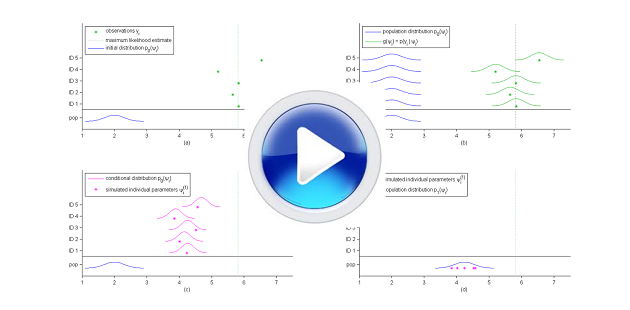# Animations & Videos

## Introduction to the population approach

The goal of this animation is to show that the population approach is relevant to many fields of application (biology, agronomy, toxicology, pharmacology, etc.) and to present a PK modeling application in a bit more detail.## Introduction to PK modeling

This animation describes how complex biological phenomena can be approximated by simplified models represented by mathematical equations.Examples of PK modeling using \$\mlxplore\$ can be visualized here.

## The SAEM algorithm in practice

This video shows how the SAEM algorithm works, as described in The SAEM algorithm for estimating population parameters section.